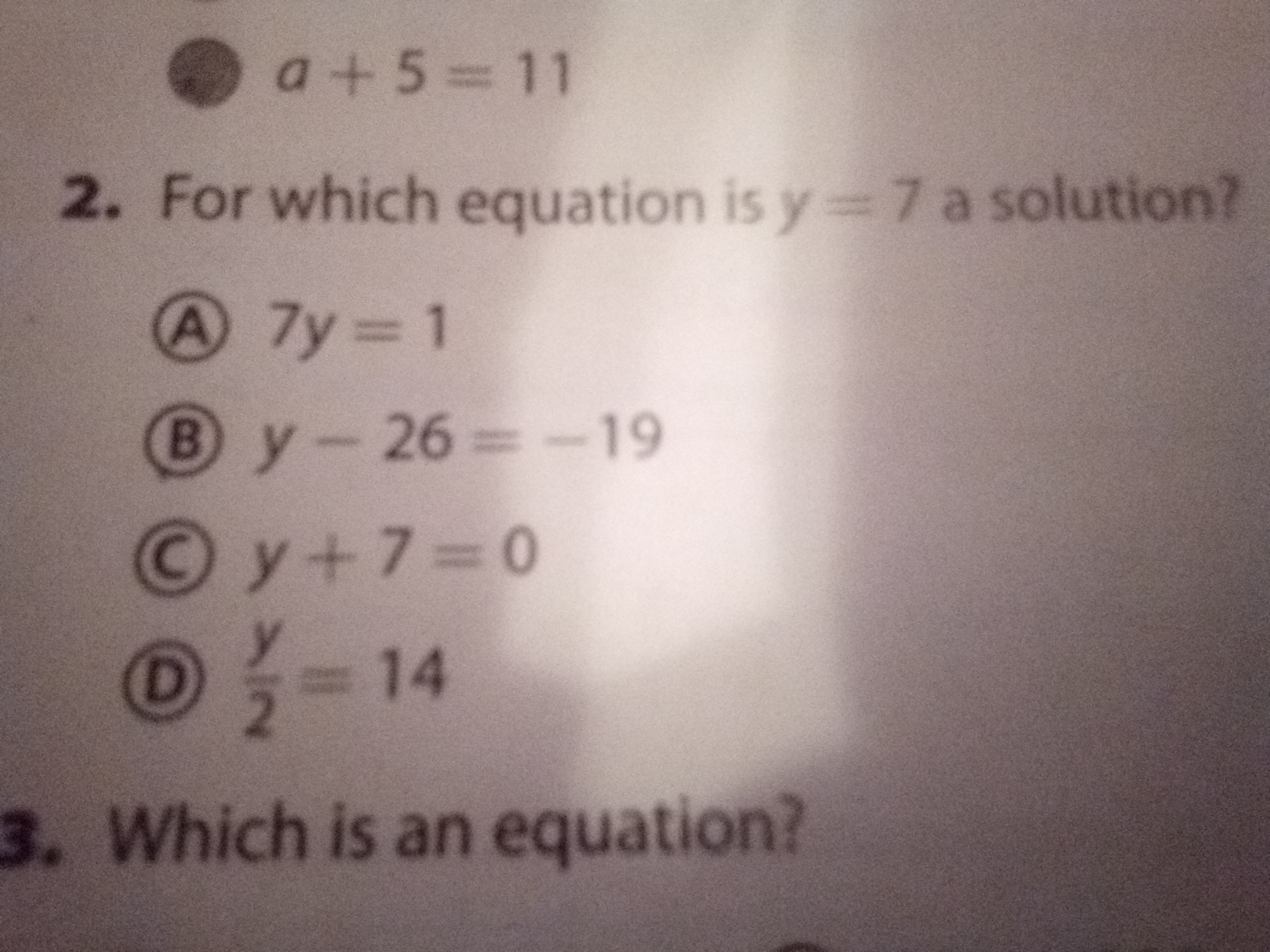# a+5=112. For which equation is y=7 a solution?A 7y 1By-26=-19Oy+7=0O- 143. Which is an equation? a+5=112. For which equation is y=7 a solution?A 7y 1By-26=-19Oy+7=0O- 143. Which is an equation?

Question
1 viewshelp_outlineImage Transcriptionclosea+5=11 2. For which equation is y=7 a solution? A 7y 1 By-26=-19 Oy+7=0 O- 14 3. Which is an equation? fullscreenhelp_outlineImage Transcriptionclosea+5=11 2. For which equation is y=7 a solution? A 7y 1 By-26=-19 Oy+7=0 O- 14 3. Which is an equation? fullscreen
check_circle

Step 1

We know that y=7 is solution of an equation if it satisfies the equation.

Step 2

We will substitute y=7 in each equation and check whether left han...

### Want to see the full answer?

See Solution

#### Want to see this answer and more?

Solutions are written by subject experts who are available 24/7. Questions are typically answered within 1 hour.*

See Solution
*Response times may vary by subject and question.
Tagged in

### Algebra